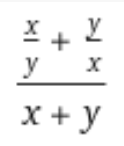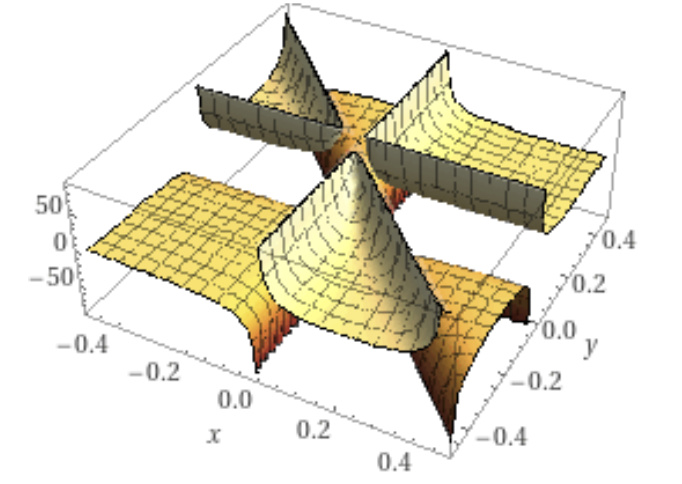# Concave Rational Function using DCP

I have checked the convexity of this functionfor 0<x<1, 0<y<1but I cannot write it using DCP rules. Any suggestion on how to express it using DCP rules?

I also checked the concavity, and found it to be neither convex nor concave, even when restricted to 0 < x < 1, 0 < y < 1.

For instance, at x=0.8, y=0.2, the Hessian has eigenvalues of -0.16 and 246.06. Therefore, it can’t be used in CVX. Your only hope of using it in CVX is if the context of its use allows a reformulation without explicitly forming the function; but I doubt that is the case.

1 Like

Thanks @Mark_L_Stone ! Why do you think the plot shows convexity (I have generated that from wolfram alpha) for x,y in that interval but the Hessian evaluated at that point is not PSD?

I don’t know. Determining concavity from 3D plots is outside my expertise. it is also not an approved method per the link I provided.

It actually is convex for a large portion of 0 < x < 1, 0 < y < 1. I am not aware of any point in that region at which it is concave,

1 Like

The plot is not very precise in the area you need. Plug in Mark’s `y=0.2` and plot the function of one variable `x`, then the problem is clear.

1 Like